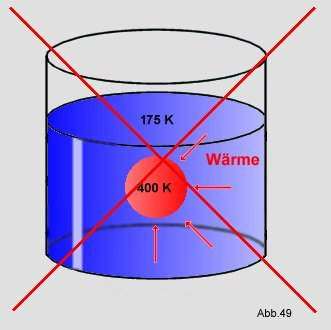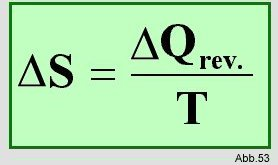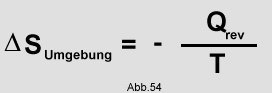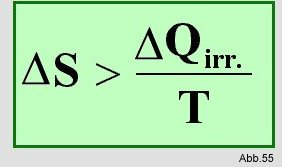# What is entropy in thermodynamics 2

Page - 21 -

IV. The Laws of Thermodynamics

### = Entropy law of thermodynamics

The first law sets limits through the conservation of energy within which a process must take place. However, there are processes that would not violate the law of conservation of energy, but do not occur in nature. For example, if you put a 300K warm ball in a vessel with 275K cold water, heat flows to the water until both have the same temperature:But it has never happened before that heat flows from colder to warmer, which would increase the temperature difference even more. The 300K warm ball is not heated to 400K, while the water should cool down to 175:As another example, consider a ball of dough falling on a board:Fig 50

The ball heats up as a result of the impact (from 293 K to 298 K).
But it has never happened before that a ball of dough suddenly jumps up while cooling:Fig 51

But that would not contradict the first law, since it only states that the sum of the energies must always be the same, and that would be it with such a process.
In order to be able to make a statement about the direction in which a process is taking place, an equation is required that only relates to the initial state of a system and the constraint exerted on it. Isolated systems always strive for equilibrium. One of the variables must be the driving force. In 1850 it was called the entropy S by Clausius. The 1st law describes the energy function U and the 2nd the entropy function. Just like the internal energy U, the 2nd law is also a state function.

The total entropy in an isolated system can never decrease, i.e. it can only increase or remain the same. A system can no longer change when the entropy has reached its maximum value, the system is then in equilibrium.
In a closed system that is in equilibrium:Such a reaction must take place with total reversibility. Total reversibility means that the reaction must proceed infinitely slowly so that the same temperature and pressure always prevail everywhere. For example, gas molecules in a gas that is being expanded whirl around and create turbulence, so that the exact reversal of this process is impossible. If, on the other hand, one expands infinitely slowly, so that no turbulence or the like forms, then the reversal is more possible.
This shows that a reversible reaction is an ideal case, which is sometimes almost reached, but in reality can never be completely reached.
Theoretically, however, the reversibility is treated. It is said that in a closed system in which the temperature and pressure remain the same, a spontaneous reversible process has the following change in entropy:At the temperature T, the system takes the amount of heat Qrev. (= Amount of heat in a reversible process).
It follows that the change in entropy of the environment is defined as follows:All processes can be classified either as reversible or as irreversible. Irreversible means that the exact course of the process can no longer be exactly reversed. In these processes, the entropy must always increase, which is why its change is defined as follows:In a closed system, the sum of the entropies of the system and the environment must be less than 0:The 2nd law describes the direction of energy conversion. If you z. If, for example, a tightly closed container is considered in which two gases are enclosed, then these gases will mix evenly over time, i.e. they will assume a state of greater disorder and thus greater entropy. So the state of greater entropy is the more likely state. From the 2nd law it follows that heat cannot be transferred from an area with a lower temperature to an area with a higher temperature if no work is done.

Perpetual motion machine:
The 2nd law also has a meaning for thermodynamics. A machine that would do work in violation of the 2nd law is called a 'perpetual motion machine of the 2nd kind'. That would be, for example, a heat engine that could extract heat from a cold environment in order to do work in a warm environment. Loud However, this is impossible according to the 2nd law, so a machine that, for example, converts the heat reservoir of the oceans into work cannot exist.
Planck suggested this fact "the prohibition of the perpetual motion machine 2nd kind" to call.

Historical quotes:

The scientist Sir Kelvin put it:
"It is impossible to design a cycling machine that has no effect other than extracting heat from a container and doing an equal amount of work."

R.J.E. Clausius, 1854, theorem on the increase in entropy:
"Entropy increases with every natural process."
Also comes from him:
"It is not possible to construct a cyclically working machine that produces no other effect than the transfer of heat from a colder to a warmer body."
and its summary of the 1st and 2nd law:
"The energy of the universe is constant, the entropy of the universe always strives to a maximum, the 1st law is the law of conservation of energy and therefore a law of symmetry. The 2nd is not a law of symmetry and it expresses a principle that breaks the symmetry of the universe by it demands a certain direction of its change. "